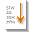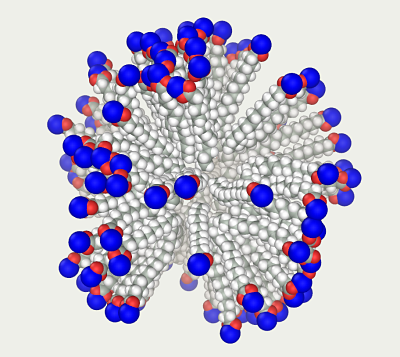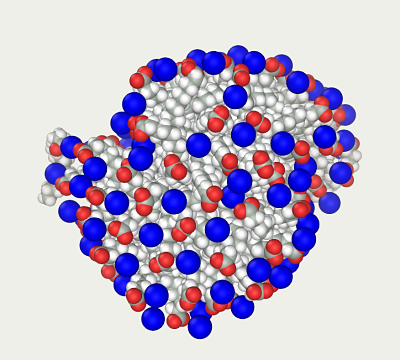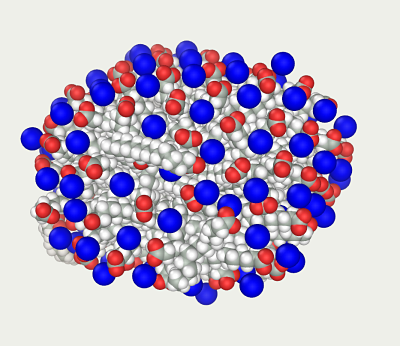# Scripts

Abalone supports the JavaScript language (to be precise, the ECMAScript standard). Unlike other software where scripting is the main way to control the program, scripting is an optional interface here. Abalone was designed as a graphical system where molecules are typically manipulated with a mouse. Scripting in Abalone is largely intended for:

## Initial Steps

The Script panel is invoked from the Compute>Script menu or by pressing the toolbar icon. The number of such panels is not limited, and they operate independently driven by their own ScriptEngines. The script text can be entered manually or loaded from a file.

The standard Info panel is used for text output. A text is displayed in the Info panel

• As a return value of the script. Commonly, it is a result of the last function in the script or an error message. For example, enter 2*2 to the script panel and press the Run button. The result of the arithmetic expression appears in the Info panel;
• After a text is entered into the global info object. Execute 'tutor 001 info.js'. This script demonstrates two main Info methods: clear() and append(). These methods suffice for most tasks. The complete description of the info methods can be found in the Qt documentation. Info corresponds to the QTextBrowser class of objects.

Each script initially contains several objects that are entry points to the system. The available objects can be accessed by applying the JavaScript operators for ... in to the this operator at the global level ('tutor 002.1 list Global properties.js').

``````
for (property in this)
{
info.append (property);
}
``````

One can see the window, info, random, project, property (the latter object appeared as a result of our code), etc. objects.

Thus, the basic objects of the system are: info, for information output; window, to control the graphical system; project, to control manipulations with molecular models; random, a Mersenne Twister random number generator.

The for ... in operators allow us to examine the properties of any object; for instance, their application to project ('tutor 002.2 list object properties.js') demonstrates that this object is a collection of models, including the current model displayed in the active (uppermost) graphical panel. The 'tutor 003.1 Model.js' script examines the properties of the current model: the list of methods is displayed; the methods counting atoms, and molecules are executed; and the model is shifted in space to illustrate manipulations. The 'tutor 003.2 load-save Model.js' script loads the buckminsterfullerene model from file, the water model from another file is added, and the resulting model is saved in the 'C60+H2O.mlm' file.

The tutors 004 and 005 demonstrate the manipulations with atoms and molecules. The 006 tutor explores the window object primarily showing the activation of the menu items.

After this introduction to script manipulation, let us exemplify this technique.

## Elaborate design

Constructing a micelle of palmitic acid ('Scripts\elaborate design\micelle.js').

The embedded constructors do not suffice for the purpose, since a spherical structure should be designed. The sphere diameter is taken very high to minimize the risk of overlapping, after which it is reduced into a more realistic structure using molecular dynamics.

Open the palmitic salt model ('palmitic ion.mlm'):

``````
``````

rotate the model:

``````
model.rotate (x, y, z, 0,0,0, angle);
``````

``````
``````

This results in a rough sphere. Save it in a file:

``````
model.save_as ('../Scripts/elaborate design/micelle 1 start.mlm ');
``````Below are two methods to contract the loose model into a dense sphere.

### Method 1

Switch to the graphical interface, since the script has made the nontrivial part of the work, and it is more convenient to do the rest manually.

The model is rather large to be calculated fast so the GPU mode should be switched on if available.

A centripetal acceleration is imposed to each molecule to contract the model into a dense sphere. This can be done by placing an atom of the dummy element CNTR with a great epsilon (e.g., 1e18 kcal/mol) into the sphere's center. Set the corresponding value in the '\data\ForceField\AMBER94_5_Van_der_Waals.xls' file:

``````
CNTR	8.	1e18	Center
``````

Add the following line to the 'micelle.mlm' file:

``````
CNTR U    0         0           0        0       CNTR             0   0  0.00    -      -        -      -
``````

Reload the file; freeze the movement of the central dummy atom; switch off the thermostat and renew velocities mode; set the temperature monitoring at each step; and start molecular dynamics. The molecules will migrate to the center. When their speed becomes high enough (e.g., the center of mass temperature reaches 1000000 K (the 'micelle 2 1e18.mlm' file), stop the dynamics and remove the dummy atom. Restarting the dynamics with constant speeds rapidly transforms the model into a dense sphere. Stop the dynamics before the molecule ends start to come in contact (the 'micelle 3 Collapse.mlm' file), otherwise the model collapses at such speeds.Now change the sodium ion radii from realistic to 5 Å. As a result, the radii of the atoms in vacuum simulations correspond to their hydration radii. In the AMBER force field, the ions with implicit hydration shells belong to the IB type; accordingly, the comment status of the following lines

``````
#Na+            Na       - IP  1.0000 IP  1.0000 IP  1.0000 IP  1.0000  -   -      -   -
Na+           Na       - IB  1.0000 IB  1.0000 IB  1.0000 IB  1.0000  -   -      -   -
``````

should be swapped in the 'FineType.mlm' file. The program should be restarted for the changes to take effect.

Eliminate the overlapping of the enlarged ions (menu Build/Remove clashes).

The model is near the local minimum; however, it is far from equilibrium configuration: sodium ions are exposed to strong electric forces so that molecular dynamics will rapidly increase their temperature to thousands of C°. A highly efficient thermostat is required to balance the system such as the Hybrid Monte Carlo method, which resets the speeds of all items at each step. Within 100 ps, the ions acquire more or less sensible position ('micelle 5 100 ps HMC.mlm') so that they do not overheat too much during the subsequent dynamics. This allows us to switch to the common dynamics with the Nose-Hoover thermostat. After 1 ns, the classical dynamics results in a realistic looking micelle ('micelle 7 1 ns MD.mlm').### Method 2

Set the sodium ion type to IB as described for the method 1 above.

Eliminate possible overlapping of the atoms.

In order to contract the model into a compact sphere, create a box (menu Settings/Boundary conditions/Periodic Box). Specify the box size twice the sphere diameter (600 Å) so that the sphere is not deformed by the interaction with the neighboring boxes. Start molecular dynamics at low temperature (Berendsen thermostat, 50 K), high pressure (100000 atm, Tau 1 ps), low cutoff (6 Å), and high accuracy of integration (1 fs step). The barostat not only changes the box size but also shifts all atoms, which draws all molecules together rapidly and low temperature suppresses their disorientation. Stop the dynamics when the molecule ends come into collision. In this case, the pressure reached 2000 atm and the center of mass temperature corresponds to 3600 K ('second micelle 2 Collapse.mlm').

Dismiss the periodic boundary conditions and cutoff. Set the normal temperature and run 100 ps of hybrid Monte Carlo dynamics followed by 1 ns of classical dynamics.

The result is quite similar to that produced by method 1 ('second micelle 3 Final.mlm').## Standard protocols

Most simulations follow the same pattern:

• constructing a raw model;
• recasting it in a realistic initial state commonly through optimization or dynamics;
• dynamics targeted to an equilibrium state;
• productive dynamics making possible the model properties to the monitored.

These manipulations or their parts can be conveniently organized into standard protocols. Let us write a realistic protocol for the equilibration stage ('equilibration.js').

Open a cubic box with water and type in the following protocol:

``````
var model = project.current_model();
var MD = model.MD();

MD.set_duration(80); // ps
MD.set_thermostat ('Berendsen');
MD.run();

MD.set_duration(40); // ps
MD.set_thermostat ('Lowe');
MD.run();

MD.set_duration(80); // ps
MD.set_thermostat ('Nose-Hoover');
MD.run();
``````

Use menu to open the molecular dynamics panel, set the thermostat type and simulation duration, and execute each stage.

This is a typical sequence of actions for model equilibration. We applied it to a water model; however, the protocol itself contains no model-specific data and just processes the current model. Accordingly, it can be applied to any model.

## Batch processing

Protein threading is perfectly suited for the script language, since scripts work well with multiple iterations and string operations.

The problem is to determine the three-dimensional structure of a protein from its amino acid sequence. One of approaches to a relatively realistic secondary structure relies on the assumption that it is determined by the interaction of the neighboring residues in the chain. Although it is not exactly correct, this approach yields correct results in many cases. Protein threading is one of popular procedures of this kind. Idealized three-dimensional templates are generated, and each of them is tried with the fragments of the studied amino acid sequence from end to end with a single amino acid shift ("threaded"). At each step, the fitness of actual sequence to the template is evaluated, commonly using the energy function so that low energy value suggests that the tested protein fragment corresponds to the template structure.

We will use a protein with known three-dimensional structure (first 35 amino acids of ubiquitin) to thread it through the templates of alpha and beta structures. Use Chain Builder to generate a structure from templates. In the script language, Chain Builder is invoked by the sequence method:

``````
var editor = model.sequence();
``````

### Alpha helix template

We will generate helices of 10 residues, which correspond to three alpha helical turns. Below is an example of 10 alanine residues:

``````
build_alpha ('AAAAAAAAAA')

function build_alpha (seq)
{
var model = project.current_model();
var editor = model.sequence();
editor.set_conformational_type ('Alpha helix');
editor.build (seq);
}
``````

### Beta sheet template

Our beta sheets consist of two stretches connected by a turn region:

``````
function build_turn (seq)
{
var model = project.current_model();
var editor = model.sequence();
editor.set_conformational_type ('II strand'); editor.build (seq.substring (0, 3));
editor.set_conformational_type ('II turn');   editor.build (seq.substring (3, 7));
editor.set_conformational_type ('II strand'); editor.build (seq.substring (7, 10));
}
``````

Warning: since vacuum simulations are performed, switch on the implicit water mode.

At each step, alpha and beta structures are generated from templates; atom overlapping is eliminated; a short dynamics is run to find a more optimal conformation followed by an optimization to find a local minimum; and the energy is calculated and saved. Thus, we obtain a table of energies for all substrings in the alpha and beta conformations.

The full script is available in the 'Scripts\batch processing\protein threading.js' file.

# JavaScript interface

## Global objects

• project
• info
• window
• pause
• random

And for use in monitors only:

• monitor_model

## Main methods

``````
project
int                 model_count   ();
model               model         (int i);
model               current_model ();
``````
``````
info
clear         ();
append        (string);
``````
``````
pause
millisecond   (int ms);
``````
``````
random
double              inc     ();          // number in [0,1]
double              inc     (double n);  // number in [0,n]
double              exc     ();          // number in [0,1)
double              exc     (double n);  // number in [0,n)
double              dbl_exc ();          // number in (0,1)
double              dbl_exc (double n);  // number in (0,n)
``````
``````
model
erase               erase ();

invert_selection ();
clear_selection  ();

int                 atom_count ();
atom                atom       (int i);

int                 bond_count ();
bond                bond       (int i);

int                 molecule_count ();
molecule            molecule       (int i);

move   (double x, double y, double z);
rotate (double around_x, double around_y, double around_z,
double point_x,  double point_y,  double point_z,  double teta);

string              name    ();
bool                save_as (string file_name);
pull_on (string file_name);

interaction         interaction ();
set_force_field (string ff);
MD                  MD ();
optimize            optimize ();
REMD                REMD ();
monitor             monitor (string name);
sequence            sequence ();
geometry            geometry ();
build               build    ();
fit_to_screen ();
``````
``````
atom
bool                is (atom);
bool                is_backbone ();

select (bool on);
bool                is_selected ();
bool                is_picked   ();
bool                is_frozen   ();

bool                has_mm_type ();
QString             mm_type     ();
set_mm_type (QString str);

bool                has_fine_type ();
QString             fine_type     ();
set_fine_type (QString name);

double              charge ();
void                set_charge (double charge);

string              element ();
set_element (string);

double              x ();
double              y ();
double              z ();

double              velocity_x ();
double              velocity_y ();
double              velocity_z ();

set_x (double x);
set_y (double y);
set_z (double z);

set_color (int R, int G, int B);
set_style (string);             // CPK, stick, ball_and_stick,
// wireframe or wireframe_CPK

int                 bound_count ();
atom                bound_atom  (int i);
bond                bond        (int i);
bool                bound_with  (atom);
``````
``````
bond
bool                is (bond);
int                 atom_count ();
atom                atom (int i);

set_color (int R, int G, int B);
set_style (string);             // CPK, stick, ball_and_stick,
// wireframe or wireframe_CPK
``````
``````
residue
``````
``````
molecule
int                 atom_count ();
atom                atom (int i);

double              mass ();
double              charge ();

string              molecular_formula (string format = "ASCII"); // Format ASCII or HTML

move   (double x, double y, double z);

rotate (double around_x, double around_y, double around_z,
double point_x,  double point_y,  double point_z,  double teta);

disorient ();
``````
``````
interaction
double              potential   ();
double              intermolecular_energy ();

zero_forces ();

zero_virial ();
double              virial      ();

set_implicit_water  (bool on);
set_method          (string method); // "Sheffield" or "Generalized Born"

set_reduced_charges (double k);
``````
``````
MD
run ();

set_duration            (double ps);
set_desired_temperature (double K);
set_desired_pressure    (double atm);

set_thermostat          (string name);
``````
``````
optimize
run ();
``````
``````
REMD
set_mode (string mode); // "Replica exchange". "Parallel dynamics"
set_temperature_from (double T);
set_temperature_to   (double T);
replicate            (int replica);

set_exchange_per_step (int);
set_attempts          (int);
set_steps             (int);
run ();
``````
``````
monitor
double              last_value ();
double              average    ();
double              rmsd       ();
``````
``````
sequence
change_repository       (string);
set_chain_type          (string);
set_conformational_type (string);
build                   (string);
``````
``````
geometry
double              distance           (int atom1, int atom2);
set_distance       (int atom1, int atom2, double value);
add_distance       (int atom1, int atom2, double value);

double              angle              (int atom1, int atom2, int atom3);
set_angle          (int atom1, int atom2, int atom3, double value);
add_angle          (int atom1, int atom2, int atom3, double value);

double              dihedral_angle     (int atom1, int atom2, int atom3, int atom4);
set_dihedral_angle (int atom1, int atom2, int atom3, int atom4, double value);
add_dihedral_angle (int atom1, int atom2, int atom3, int atom4, double value);
``````
``````
build
atom                new_atom           (string element);
bond                new_bond           (atom, atom, string order); // order can be Single,
// Double, Triple, or Aromatic
``````
``````
erase
add (object); // object can be atom, bond, molecule, residue or interaction
execute ();
``````